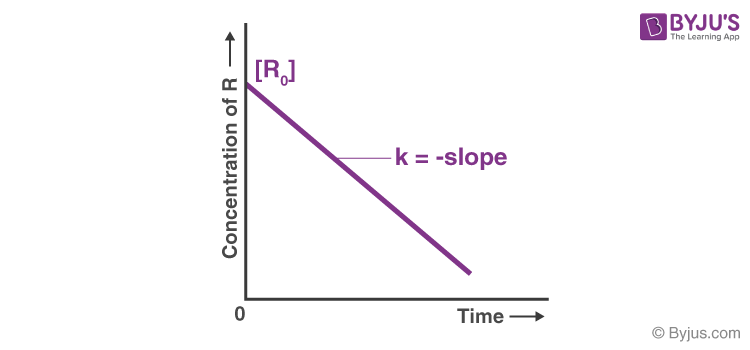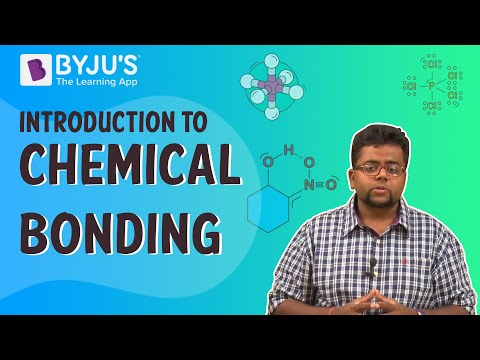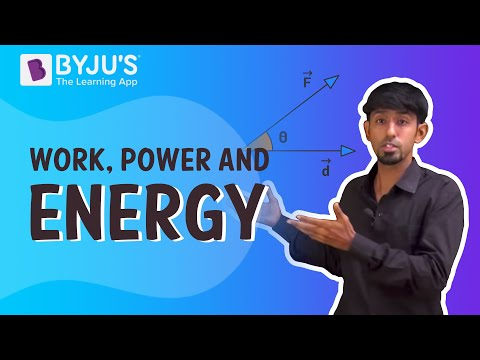# Chemistry Important Questions

Chemistry is the study of the properties and behaviour of matter. It covers the composition of matter, structure, properties of substances, the transformations that matter undergoes, and the energy released or absorbed during these processes. We can further classify chemistry into five types, i.e. inorganic chemistry, organic chemistry, physical chemistry, analytical chemistry and biochemistry.

 Definition: Chemistry is the branch of science that deals with the composition, structure, properties of substances, the transformations that matter undergo, and the energy that is released or absorbed during these processes.

## Chemistry Important Questions with Solutions

Q1. Which ionic compound shows both frenkel and schottky defects?

1. AgBr
2. NaCl
3. KCl
4. CsBr

Answer: (a) AgBr shows both frenkel and schottky defects.

Explanation: AgBr shows both frenkel and schottky defects because the radius of silver bromide is intermediate. It illustrates the schottky defect when the cation and anion are absent from the crystal lattice. In contrast, it reflects a frenkel defect when the Ag+ ions leave their original place in the crystal lattice to create a vacancy while occupying an interstitial position.

Q2. Which among the following is produced when we pass chlorine into slaked lime?

1. DDT
2. Bleaching Powder
3. Gypsum
4. Baking Powder

Answer: (b) Bleaching Powder is produced when we pass chlorine into slaked lime.

Explanation: When chlorine is passed over slaked lime, Ca(OH)2, it is rapidly absorbed, forming bleaching powder or chloride of lime.

The reaction is: Ca(OH)2 + Cl2 → CaOCl2 + H2O

Q4. Fluorine does not exhibit any positive oxidation state. Why?

Answer: Fluorine is the most electronegative element in the periodic table. For ascertaining a positive oxidation state, it should be bonded to a more electronegative element than fluorine. But there is no such element in the periodic table. Hence, fluorine always shows a negative oxidation state and can not ascertain a positive oxidation state.

Q5. What is pyrometallurgy?

Answer: Pyrometallurgy is the branch of metallurgy that deals with the extraction and purification of metals by heat processes. Roasting, Smelting and Refining are the three primary pyrometallurgical operations.

Q6. What is Tollen’s reagent? Write one use of this reagent.

Answer: Ammonical silver nitrate solution (AgNO3 + NH3OH) is known as Tollen’s reagent. It is used to witness −the CHO group in an organic compound.

Q7. What are the differences between order and molecularity of reaction?

S. No.

Order of reaction

Molecularity of reaction

1.

Order is the sum of the exponents of concentrations in the rate law equation.

Molecularity is the number of atoms, molecules or ions that must collide in a chemical reaction.

2.

Order of reaction can be fractional or zero.

The Molecularity of reaction is always a natural number.

3.

It is determined experimentally.

It can be calculated by adding molecules of the slowest step.

Q8. For a chemical reaction R → P, the variation in concentration vs time plot is given as(i) Predict the reaction order.

(ii) What is the slope of the curve?

Answer: (i) It is a zero-order reaction.

(ii) Slope of the curve = -K

Q9. The temperature on the Celsius scale is 25o C. What is the corresponding temperature on the Fahrenheit scale?

Answer: T(o C) = 25o C

We know that,

T(o F) = T(o C) X 9 / 5 + 32

T(o F) = 25 X 9 / 5 + 32

T(o F) = 77o F

Q10. What is aldol condensation?

Answer: ‘Aldol’ is the abbreviation of aldehyde and ketone. In it, dimerisation of aldehyde or ketone occurs. An enolate ion reacts with a carbonyl compound to form a beta-hydroxy aldehyde or beta-hydroxy ketone, escorted by eliminating water molecules to yield a conjugated enone.

CH3-CHO + CH3-CHO → CH3-CH(OH)-CH2-CHO

Q11. The pH of a solution of a strong acid is 5.0. What will be the pH of the solution obtained after diluting the given solution 100 times?

Answer: pH = 5 means [H+] = 10−5

On diluting 100 times ,

[H+] = 10−5 / 100 = 10−7

On calculating the pH using the equation pH = − log [H+], the pH value comes out to be 7, which is impossible.

Hence, Total H+ ion concentration = H+ ions from acid + H+ ion from water

[H+] = 10−7 +10−7 M

[H+] = 2 X 10−7

pH = 7 – 0.3010

pH = 6.699

Q12. What is the molarity of a solution prepared by dissolving 4g of NaOH in 3L of the solution?

Answer: Weight of NaOH= 4 g

Molecular mass of NaOH= 40 g

Volume of solution =3L

Molarity can be calculated by

Molarity = Mass of solute / Molarmass of solute X Volume in l

Molarity = 5 / 40 X 3

Molarity = 5 / 120

Molarity = 0.04M

Hence, the molarity of a solution prepared by dissolving 4g of NaOH in 3L of the solution is 0.04M.

Q13. Calculate the number of unit cells in 8.1 g of aluminium if it crystallizes in an fcc structure. ( Gien, Atomic mass of Al = 27 g mol-1)

Mass of Al = 8.1,

Atomic mass of Al = 27 g mol-1

No. of atoms = η × 6.022 × 1023

= 8.1 / 27 × 6.022 × 1023

= 0.3 × 6.022 × 1023

= 1.8066 × 1023

Since one fcc unit cell has 4 atoms

∴ No. of unit cells = 1.8066 × 1023/ 4

= 4.5 × 1022 unit cells

Q14. What are the differences between an ideal solution and a non-ideal solution?

S. No.

Ideal Solution

Non-Ideal Solution

1.

They obey Raoult’s law over the entire range of concentration.

They don’t obey Raoult’s law over the entire range of concentration.

2.

Their vapour pressure lies between the vapour pressure of pure components.

Their vapour pressure doesn’t lie between the vapour pressure of pure components.

3.

Example: Benzene, Toluene.

Example: Ethanol, Chloroform, Acetone.

Q15. Match the statement given in Column I with the phenomenon given in Column II.

Column I

Column II

(i) Dispersion medium moves in an electric field

(a) Osmosis

(ii) Solvent molecules pass through a semi-permeable membrane towards the solvent side

(b) Electrophoresis

(iii) Movement of charged colloidal particles under the influence of applied electric potential towards oppositely charged electrodes

(c) Electroosmosis

(iv) Solvent molecules pass through semi-permeable membranes towards solution side

(d) Reverse osmosis

## Practise Questions on Chemistry

Q1. The graph between log (x / m) and log p is a straight line at an angle of 45 with an intercept on the y-axis of 0,3010. Calculate the amount of gas adsorbed in gram per gram of the adsorbent when pressure is 0.2 atm.

Q2. What is the molarity and normality of a MnO4 solution if 32mL of the solution is required to titrate 40mL of 0.4 N Fe2+.

MnO4 + 5Fe2+ + 8H+ → Mn2+ + 5Fe3+ + 4H2O

Q3. A 250mL sample of 0.20M hydrochloric acid is to be made by diluting the approximate amount of the concentrated reagent 11.7M. What volume of the latter should be used?

Q4. A carbon compound with acidic characteristics is used as a preservative for pickles. This compound reacts with carbonates and bicarbonates to release a colourless gas. Identify the compound and the gas. Give the equations for the reactions also.

Q5. The compound ‘A’ with an excess of isopropyl magnesium iodide, upon hydrolysis, gives tertiary alcohol. Identify A.

Click the PDF to check the answers for Practice Questions.

## Recommended Videos

#### Introduction to Chemical Bonding